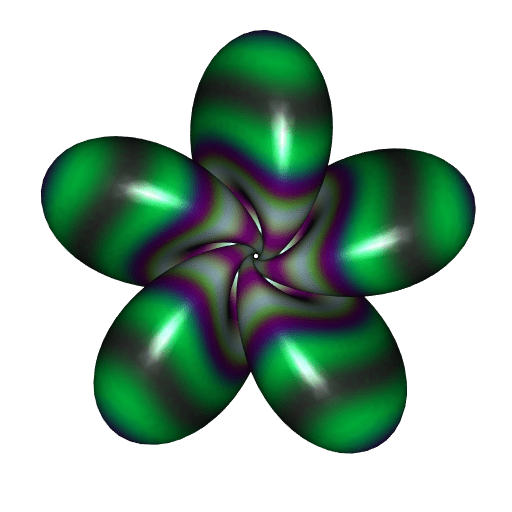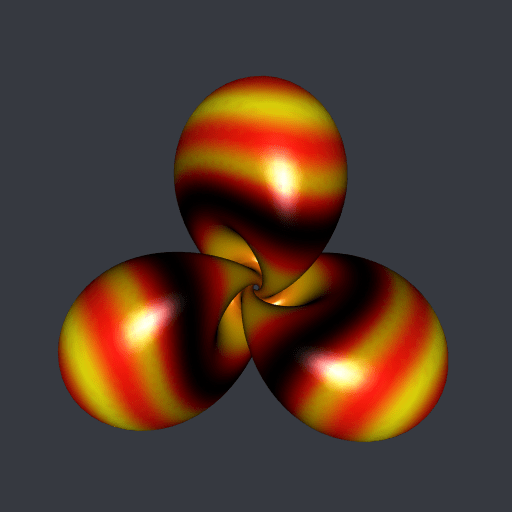[This article was first published on Saturn Elephant, and kindly contributed to R-bloggers]. (You can report issue about the content on this page here)
Want to share your content on R-bloggers? click here if you have a blog, or here if you don't.

In a recent post I explained how to decorate a surface with moving colors with the Python library PyVista. Here I expose this method for the R package rgl.

I will take a Hopf torus with fives lobes for the illustration. The following R function is a slight modification of the parametric3d function of the misc3d package. It is more efficient for the Hopf torus parameterization and it returns a rgl object of class mesh3d.

library(misc3d)
library(rgl)

parametricMesh3d <- function(
Fxyz, umin, umax, vmin, vmax, nu, nv
){
u <- seq(umin, umax, length.out = nu)
v <- seq(vmin, vmax, length.out = nv)
tg <- misc3d:::expandTriangleGrid(u, v)
f <- function(uv) Fxyz(uv[, 1L], uv[, 2L])
v1 <- f(tg$v1) v2 <- f(tg$v2)
v3 <- f(tg$v3) tris <- makeTriangles(v1, v2, v3) mesh0 <- misc3d:::t2ve(tris) addNormals( tmesh3d( vertices = mesh0$vb,
indices  = mesh0$ib ) ) } The R function below is the parameterization of the Hopf torus. HTxyz <- function(u, v, nlobes = 5, A = 0.38){ C <- pi/2 - (pi/2-A)*cos(u*nlobes) sinC <- sin(C) D <- u + A*sin(2*u*nlobes) p1 <- cos(C) + 1 p2 <- sinC * cos(D) p3 <- sinC * sin(D) cos_v <- cos(v) sin_v <- sin(v) x1 <- cos_v*p3 + sin_v*p2 x2 <- cos_v*p2 - sin_v*p3 x3 <- sin_v * p1 xden <- sqrt(2*p1) - cos_v*p1 cbind(x1/xden, x2/xden, x3/xden) } Let’s make the Hopf torus mesh now. mesh <- parametricMesh3d( HTxyz, umin = 0, umax = 2*pi, vmin = -pi, vmax = pi, nu = 600, nv = 400 ) We will assign a color to each point on the surface, according to the distance from the point to the origin. We calculate these distances below, and we linearly map them to the interval$[0, 2].

d <- sqrt(apply(mesh\$vb[-4L, ], 2L, crossprod))
d <- 2*pi * (d - min(d)) / diff(range(d))

Now we introduce a color palette function. The trekcolors package has nice color palettes. I’m taking the dominion palette.

library(trekcolors)
fpalette <- colorRamp(
trek_pal("dominion"), bias = 0.6, interpolate = "spline"
)

This function fpalette assign a color, given by its RGB values, to each number between $$0$$ and $$1$$. As in Python, we will calculate $$\sin(d -t)$$ to move the colors, with $$t$$ varying from $$0$$ to $$2\pi$$. The sine function takes its values in $$[-1, 1]$$ so we will map this interval to $$[0,1]$$ with the affine function $$x \mapsto \frac{x+1}{2}$$ in order to apply the fpalette function.

We will also rotate the Hopf torus around the $$z$$-axis. By the symmetry of the Hopf torus, it suffices to make the rotation with an angle varying from $$0$$ to $$2\pi/5$$.

t_ <- seq(0, 2*pi, length.out = 73)[-1L]
angle_ <- seq(0, 2*pi/5, length.out = 73)[-1L]
for(i in seq_along(t_)){
RGB <- fpalette( (sin(d-t_[i])+1)/2 )
mesh[["material"]] <-
list(color = rgb(RGB[, 1L], RGB[, 2L], RGB[, 3L], maxColorValue = 255))
rmesh <- rotate3d(mesh, angle_[i], 0, 0, 1)
open3d(windowRect = c(50, 50, 562, 562))
view3d(0, 0, zoom = 0.55)
spheres3d(0, 0, 0, radius = 11, color = "white", alpha = 0) # to fix the view
rgl.snapshot(sprintf("pic%03d.png", i))
close3d()
}

This code generates a series of png files pic001.png, …, pic072.png. Using ImageMagick or gifski, we obtain this gif animation from these files:Very well. But these are not my favorite colors. And I prefer the Hopf torus with three lobes. Below it is, decorated with the klingon color palette of trekcolors; I prefer this one.